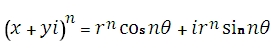# SQL Server IMPOWER function

Updated: 27 August 2010

Use IMPOWER to return a complex number in x + yi or x + yj text format raised to a power. A complex number raised to a power is calculated as follows:Where
r = IMABS(x+yi)
q=IMARGUMENT(x+yi)
Syntax
SELECT [wctEngineering].[wct].[IMPOWER] (
<@inumber, nvarchar(4000),>
,<@Number, float,>)
Arguments
@inumber
is a complex number you want to raise to a power. @inumber1 is an expression of type varchar or of a type that can be implicitly converted to varchar and must be in the format x + yi or x + yj, where x is a real number that can be converted to float and yi (or yj) is an imaginary number, where the y part can be converted to float.
@Number
is the power to which you want to raise the complex number. @Number is an expression of type float or of a type that can be implicitly converted to float.
Return Types
varchar(4000)
Remarks
·         Use COMPLEX to convert real and imaginary coefficients into a complex number
Examples

select
wct.IMPOWER('2+3i', 2)

This produces the following result
-------------------------
-5+12i

(1 row(s) affected)

### SupportCopyright 2008-2023 Westclintech LLC         Privacy Policy        Terms of Service